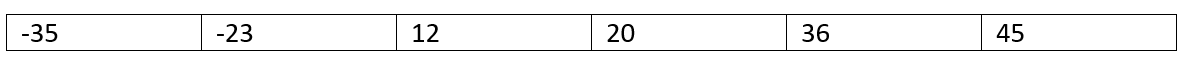# Two Elements whose sum is closest to zero

Here, we are going to learn how to find two elements out of an array whose sum is closest to zero using C++?
Submitted by Radib Kar, on January 27, 2021

For example,

You are given an array [-23, 12, -35, 45, 20, 36] then the two elements would be -35 & 36 as their pair sum is 1 which is closest to 0. You can generate all the pair sums and validate yourself.

The naïve way of solving this is by finding all the pair sum. Of Couse, this will require two loops and will take O(n^2) time. Below is the simple implementation for the above naïve approach.

```for(int i=0;i<arr.size();i++){
for(int j=0;j<arr.size();j++){
if(i!=j && abs(arr[i]+arr[j]) < abs(closestSum)){
number1=arr[i];
number2=arr[j];
closestSum = arr[i]+arr[j] ;
}
}
}
```

The efficient way is two pointer approach. The idea is to sort the array. For example, if we sort the above array, we will haveNow set the two pointer, one at the beginning and another at the end. Now, keep calculating the sum and move the pointers as done in the below code,

```int start =0, end = arr.size()-1;
int number1=0,number2=0;
while(start<end){
//when sum is 0 itself
if(arr[start]+arr[end]==0){
number1=arr[start];
number2=arr[end];
break;
}
else if(arr[start]+arr[end]>0){
if(abs(arr[start]+arr[end]) < abs(closestSum) ){
number1=arr[start];
number2=arr[end];
closestSum = arr[start]+arr[end] ;
}
end--;
}
else{
if(abs(arr[start]+arr[end]) < abs(closestSum) ){
number1=arr[start];
number2=arr[end];
closestSum = arr[start]+arr[end] ;
}
start++;
}
}
```

C++ code to find two elements whose sum is closest to zero

```#include <bits/stdc++.h>
using namespace std;

//print ceil & floor for the number
void ClosestToZeroNaive(vector<int>& arr)
{
cout << "..............Using naive search......\n";

//O(n^2) time complexity

int closestSum = INT_MAX;
int number1 = 0, number2 = 0;

for (int i = 0; i < arr.size(); i++) {
for (int j = 0; j < arr.size(); j++) {
if (i != j && abs(arr[i] + arr[j]) < abs(closestSum)) {
number1 = arr[i];
number2 = arr[j];
closestSum = arr[i] + arr[j];
}
}
}
cout << "Two elements are: " << number1 << " , " << number2 << "\n";
}

void ClosestToZeroTwoPointer(vector<int>& arr)
{
cout << "..............Using Two Pointer approach......\n";

//O(n) time complexity

int closestSum = INT_MAX;
sort(arr.begin(), arr.end());

int start = 0, end = arr.size() - 1;
int number1 = 0, number2 = 0;
while (start < end) {
//when sum is 0 itself
if (arr[start] + arr[end] == 0) {
number1 = arr[start];
number2 = arr[end];
break;
}
else if (arr[start] + arr[end] > 0) {
if (abs(arr[start] + arr[end]) < abs(closestSum)) {
number1 = arr[start];
number2 = arr[end];
closestSum = arr[start] + arr[end];
}
end--;
}
else {
if (abs(arr[start] + arr[end]) < abs(closestSum)) {
number1 = arr[start];
number2 = arr[end];
closestSum = arr[start] + arr[end];
}
start++;
}
}

cout << "Two elements are: " << number1 << " , " << number2 << "\n";
return;
}

int main()
{
cout << "Enter number of elements\n";
int n;
cin >> n;
vector<int> arr(n);
cout << "Enter the elements\n";

for (int i = 0; i < n; i++)
cin >> arr[i];

//using navive approach
ClosestToZeroNaive(arr);
//using two pointer approach
ClosestToZeroTwoPointer(arr);

return 0;
}
```

Output:

```Enter number of elements
6
Enter the elements
-12
23
-35
45
36
20
..............Using naive search......
Two elements are: -35 , 36
..............Using Two Pointer approach......
Two elements are: -35 , 36
```

Top MCQs